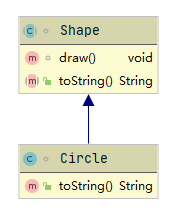# Java类型信息

运行时类型信息 ( $RTTI$ ) 可以让我们在程序运行时发现和使用类型信息，主要有两种方式：传统RTTI，假定在编译时就已经知道了所有类型；以及反射，允许在运行时发现和使用类型信息。

## 1. RTTI

传统的RTTIJava语句执行过程中也发挥着作用。我们定义了一个 $Circle$ 对象，将其存储在一 $List<Shape>$ 容器之中。那么当对象被放入容器时，会向上转型为 $Shape$ ；而在取出时，由于 $List$ 容器会将所有对象当作 $Object$ 类型持有，因此会再次转换为 $Shape$ 对象。

### 1.1 Class对象

$Class$ 对象负责表示运行时的类型信息，Java通过 $Class$ 对象执行RTTI。每个类都拥有一个 $Class$ 对象，当它被编译时，就会通过类加载器产生一个 $.class$ 文件，存储其 $Class$ 对象。
所有的类都是在被第一次使用时动态地被加载到JVM当中的。当程序创建第一个对类的静态成员的引用时，就会加载这个类。也就是说构造器也是静态的，因此使用 $new$ 创建对象就会创建一个对静态构造器的引用，从而使得这个类被加载。当类的 $Class$ 对象被载入后，这个类的所有对象都会使用 $Class$ 对象创建。$Class$ 对象还有一些常用方法：

• $forName(\ )$ ：通过全类名获取对应类的 $Class$ 对象；
• $getName(\ )$ ：获取全限定的类名；
• $getSimpleName(\ )$ ：获取不含包名的类名；
• $getInterfaces(\ )$ ：$Class$ 对象中实现的接口；
• $getSuperclass(\ )$ ：获取基类的 $Class$ 对象。

$Class.newInstance(\ )$ 方法允许你在不知道确切类型的情况下创建对象。通过该方法你可以得到一个 $Object$ 对象，要想正确地使用该对象，你需要对其进行转型。使用 $newInstance$ 创建对象的要求是该对象拥有一个默认构造器。
除了通过 $forName$ 获取 $Class$ 对象之外，还可以直接通过类字面常量获取，即访问一个类型的 $class$ 字段。而且因为这种方式在编译阶段就会受到检查，因此不需要对异常进行处理，所以也更高效。对于基本数据类型的包装类如 $Integer$ 这种，还拥有一个标准字段 $TYPE$ 。访问 $TYPE$ 就相当于访问对应基本类型的 $class$ 字段，即 $Integer.TYPE$ 相当于 $int.class$ 。
普通的 $Class$ 对象可以被重新赋值为任何其他的类型的 $Class$ 对象。而要想避免这种再次赋值，可以通过泛型进行限定。
在类型转换的过程中，Java要执行类型检查。如果执行了一个错误的类型转换，就会抛出一个 $ClassCastException$ 异常。RTTI还可以通过 $instanceof$ 进行检查，它用于判断某个对象是否是某个类型的实例，返回一个布尔值。

## 2. 反射

通过RTTI，我们可以知道某个对象的确切类型，但这是建立在编译时已知类型的前提下。如果获取了一个不在你的程序空间的对象引用，那么就无法使用RTTI获取其类型了。反射提供了一种获取对象可用方法以及方法名的机制。
$Class$ 对象与 $java.lang.reflect$ 类库一起对反射概念进行了支持，类库包含 $Field$ , $Method$ 以及 $Constructor$ 类（均实现了 $Member$ 接口），分别用于表示类里面不同类型的成员。这样我们就可以通过 $Constructor$ 创建对象，$Field$ 的 $get(\ )$ 和 $set(\ )$ 方法读取和修改字段，通过 $invoke(\ )$ 方法调用 $Method$ 对象关联的方法。这三种类型的对象分别通过 $Class$ 对象的 $getFields(\ )$ , $getMethods(\ )$ 和 $getConstructors(\ )$ 方法获取。
要获取类信息，就需要通过类的 $Class$ 对象，也即获取其 $.class$ 文件。反射与RTTI的不同之处在于，RTTI是在编译时检查 $.class$ 文件；而反射无法在编译时获取 $.class$ 文件，是在运行时检查。
虽然反射可以让我们获取类中字段、方法和构造器的信息，但它也带来了隐患：通过反射可以访问所有方法，甚至是 $private$ 方法。只需要取得方法关联的 $Method$ 对象，然后设置 $setAccessible(true)$ ，即可调用。